# An Introduction to T-Test Theory for Surveys

Learn the theory of t-tests using selected nonparametric and parametric statistical tests for two-sample cases

The T-statistic is used to test differences in the means of two groups. The grouping variable is categorical and data for the dependent variable is interval scaled. The following table shows alternative statistical techniques that can be used to analyse this type of data when different levels of measurement are available.

The t-distribution was developed by W. S. Gosset (1908)

As an employee of the Guinness brewery in Dublin, Gosset was not permitted to publish research findings in his own name, and hence penned the pseudonym “Student”. The t-distribution, as it was first designated, has been known under a variety of names, including the Student’s distribution and Student’s t-distribution.

The t-distribution revolutionised statistics and the ability to work with small samples. Prior to this time, statistical work was based largely on the value of z, which was used to designate a point on the normal distribution where population parameters were known. The z value is the deviation of the sample mean from the mean of the population and is expressed in terms of variance within a normally distributed population.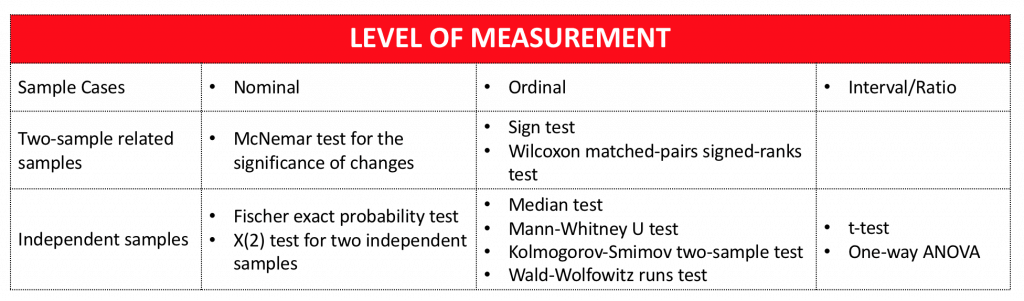The purpose of the z value is to express the amount of deviation between the sample mean and the population mean and to permit the making of inferences as to whether the sample mean belongs to the population in question. The mean and variance characteristics of the population (μ and σ), to which we desire to make inferences, are rarely known. This state of perfect knowledge is the assumption made by the z test and in actual use is difficult to justify. The t statistic does not require the population variance information needed for the z test, but instead uses the sample variance (and sample standard deviation).

The t-distribution is symmetrical about the mean and is approximately normal. It is centred at the population mean of 0 and for large samples has a variance σ = 1.

The central limit theorem tells us that the sampling distribution of all possible sample means x ̅, approaches normality as the size of the samples increase. This is true, even when the population is not normally distributed. The t-distribution rapidly approaches the shape of a normal distribution as sample size increases. The t distribution is considered normal when n=30.

The t-distribution is used to make inferences concerning the difference between the two populations μ1 and μ2. The specific statistical theory used relates to the distribution of differences between the two sets of independent sample means, the sampling distribution of x1- x2.

## Mathematical Computations for the T-Test

Statistical analysis programs compute t-statistics and associated probability levels for the equality of the means of two groups based on pooled and separate variance estimates. An F-statistic and associated probability level for the equality of group variances is also computed. Groups may be defined by specifying codes to be included. Several dependent variables may often be analysed concurrently. Paired comparison t ratios may be obtained through the use of IF and RECODE commands (SPSS).

Typical t-test computations include:

1. F-ratio of variance
2. t-value (based on pooled variance estimate)
3. t-value (based on separate variance estimate)
4. Two-tailed probability levels for each t and for the F
5. Means
6. Standard deviations
7. Standard error of the means
8. Number of observations included in computing 5-7 above

In computing the variance, it is necessary to pool variance estimates when for the two groups, sample sizes are unequal and variances are unequal (Levine’s test or O’brien’s test). In case of pooling, we pool the point estimate by simple averages.
The pooled standard error is given by:

## COMPUTATIONAL PROCEDURE

Each problem is divided into two groups: an X and a Y category. For each analysis, the number of non-missing observations, the mean, standard deviation, and standard error are computed for each variable of each category. The t-values, F-values, and corresponding probability level for between-category comparison are computed for each variable.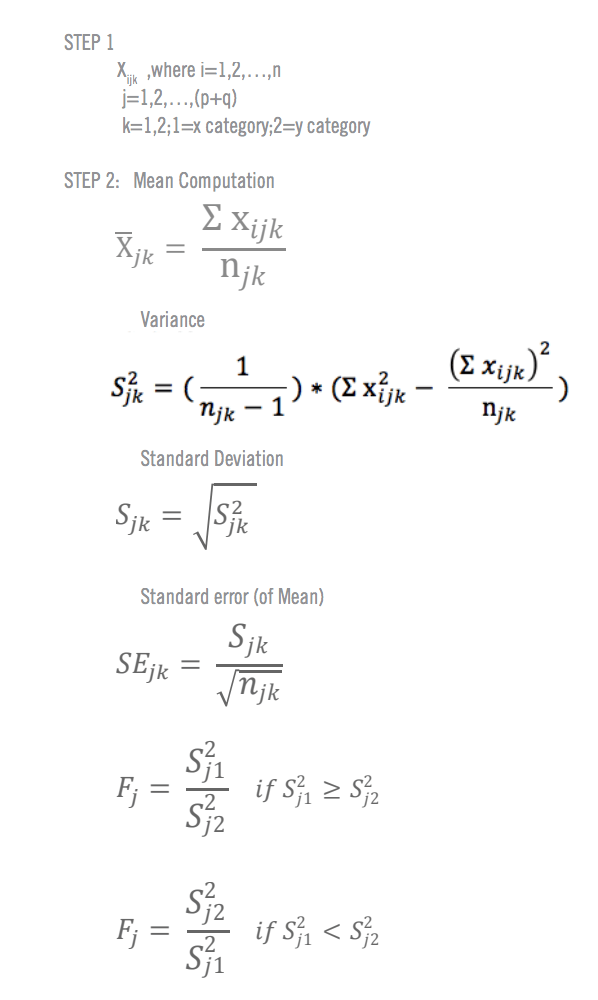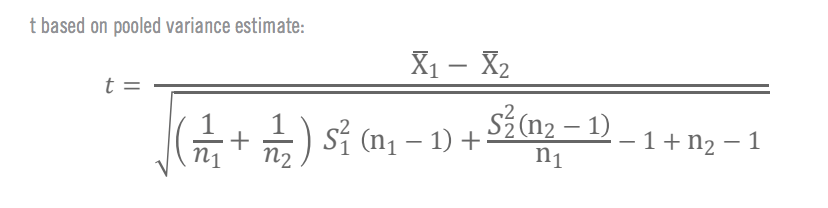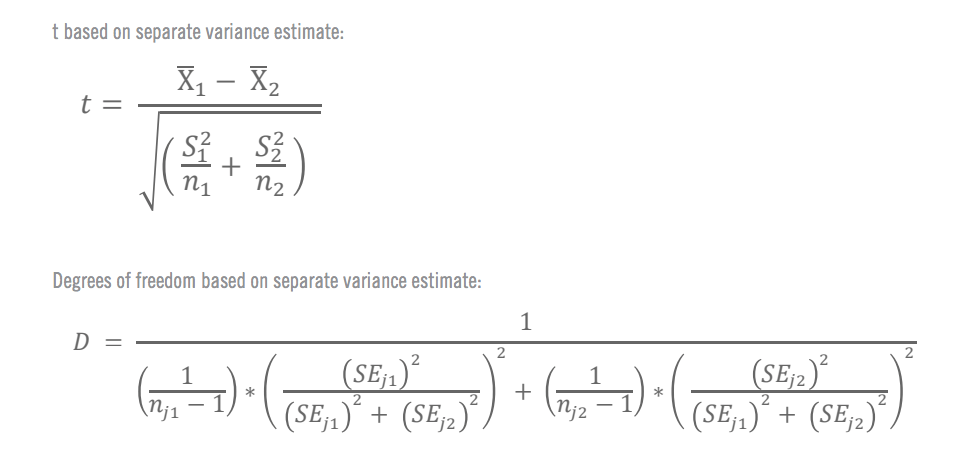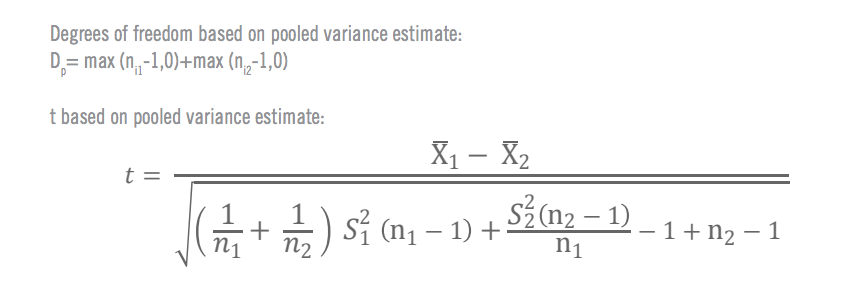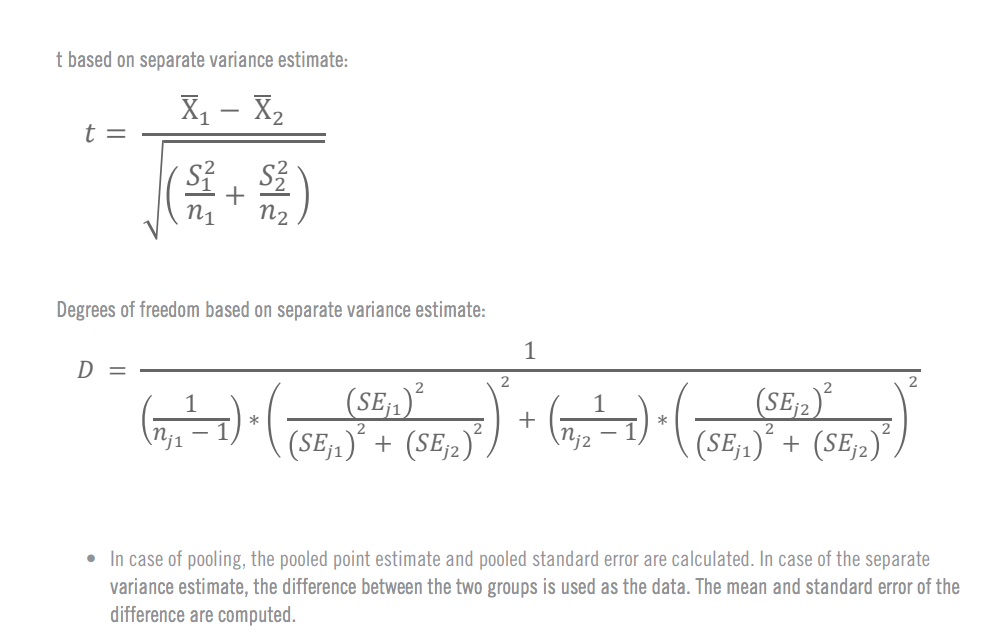Super Charge Your Survey Statistics With Qualtrics